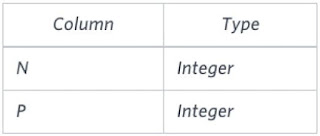# HackerRank: [SQL Advanced Select] (4/5) BINARY TREE NODES | case, when, if, sub-query in SQL

I started studying SQL from a very famous site - HackerRank. Here I will try to provide multiple approaches & solutions to the same problem. It will help you learn and understand SQL in a better way.

Please make use of my blog posts for learning purpose only and feel free to ask your questions in the comment box below in case of any doubt.

SQL Problem Statement:

You are given a table, BST, containing two columns: N and P, where N represents the value of a node in Binary Tree, and P is the parent of N.

Write a query to find the node type of Binary Tree ordered by the value of the node. Output one of the following for each node:

• Root: If node is root node.
• Leaf: If node is leaf node.
• Inner: If node is neither root nor leaf node.

Input Format:

The BST table is described as follows:BST_Columns

Sample Input:
The BST table contains the following records:
Sample Output:
```1 Leaf
2 Inner
3 Leaf
5 Root
6 Leaf
8 Inner
9 Leaf```

Explanation:
The Binary Tree below illustrates the sample:

### Solution-1: Using CASE WHEN Statement (MySQL Query):

```SELECT N,
CASE
WHEN P IS NULL THEN 'Root'
WHEN (SELECT COUNT(*) FROM BST WHERE B.N=P)>0 THEN 'Inner'
ELSE 'Leaf'
END AS PLACE
FROM BST B
ORDER BY N;```

NOTE:
1. Parent for Root Node is Null.
2. Any node which is the parent node of other nodes is an Inner node
3. All remaining nodes are Leaf nodes.

### Solution-2: Using IF Statement (MySQL Query):

```SELECT N,
IF( P IS NULL, 'Root',
IF ((SELECT COUNT(*) FROM BST WHERE B.N = P) > 0, 'Inner', 'Leaf')
) AS PLACE
FROM BST B
ORDER BY N;```

### Sample Output:

```1 Leaf
2 Inner
3 Leaf
4 Inner
5 Leaf
6 Inner
7 Leaf
8 Leaf
9 Inner
10 Leaf
11 Inner
12 Leaf
13 Inner
14 Leaf
15 Root```

--------------------------------------------------------------------------------